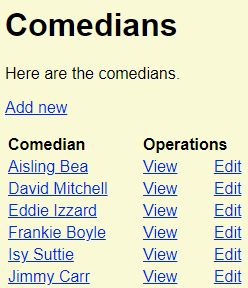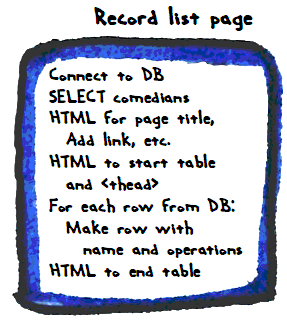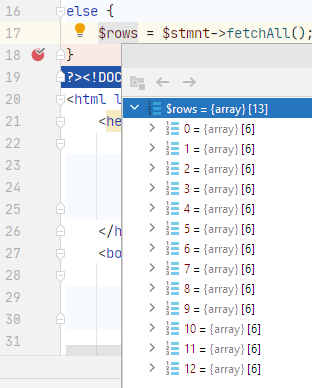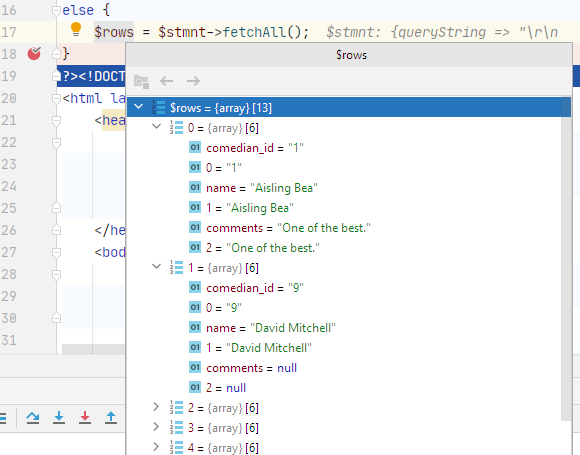# Record listMarco's Dino

We need one more component for a complete comedians management app. We need a list of comedians, with operations links. Here's what we'll make:There's an add link at the top, then a table listing each comedian. Each row has an operations column, with links to view and edit the row. This is a common way of doing things.

### HTML tables

We'll use a new HTML tag set to make the table. An HTML table is a set of rows and columns, like this:

Data Data Data
Data Data Data

Data Data Data
Data Data Data

Column headings usually have special styling, like bold text.

Several HTML tags work together to make tables. Here's an example.

1. <table>
3.     <tr>
7.     </tr>
9.   <tbody>
10.     <tr>
11.       <td>Data</td>
12.       <td>Data</td>
13.       <td>Data</td>
14.     </tr>
15.     <tr>
16.       <td>Data</td>
17.       <td>Data</td>
18.       <td>Data</td>
19.     </tr>
20.   </tbody>
21. </table>

The `table` tag wraps everything. Inside it, there are two areas: `thead`, and `tbody`. The head contains the column headings. The body has the data.

The `tr` tag makes a table row. Inside `tr`s, use `th` or `td` to make cells. Use `th` in head rows, and `td` in body rows.

### HTML for the record list

We'll write PHP that generated HTML to show the record list. Here's the list again:So we'll make HTML to show that. It helps to know what the HTML would look like:

1. <h1>Comedians</h1>
2. <p>Here are the comedians.</p>
4. <table class='record-table'>
6.     <tr>
7.       <th>Comedian</th>
8.       <th>Operations</th>
9.     </tr>
11.   <tbody>
12.     <tr>
13.         <td>
14.           <a href='view-comedian.php?comedian_id=1'>Aisling Bea</a>
15.         </td>
16.         <td class='operations-container'>
17.           <a href='view-comedian.php?comedian_id=1'>View</a>
19.         </td>
20.     </tr>
21.     <tr>
22.         <td>
23.           <a href='view-comedian.php?comedian_id=9'>David Mitchell</a>
24.         </td>
25.         <td class='operations-container'>
26.           <a href='view-comedian.php?comedian_id=9'>View</a>
28.         </td>
29.     </tr>
30.     ...
31.   </tbody>
32. </table>

We want a row for each comedian. Lines 12-20 is a row for one comedian. Lines 21-29 is the row for another. Each row has data from the DB. Lines 14 and 23 have comedian names, and ids. Rows 17 and 26 have a View link, with the comedians' ids. Rows 18 and 27 have an Edit link, with the comedians' ids.

This is what our PHP should make. Each comedian will have a table row, like lines 12-20.

### What we want to do

Let's work out what we want the code to do. Check this out.​Making the record list

• Connect to the DB.
• Make an SQL statement, to SELECT all of the comedians. Run it, get some DB rows back.
• Output HTML for the page title, Add new link, and the top of the table. This stuff:
• <h1>Comedians</h1>
• <p>Here are the comedians.</p>
• <table class='record-table'>
•     <tr>
•       <th>Comedian</th>
•       <th>Operations</th>
•     </tr>
•   <tbody>

That's done once on the page.Then, go through the data we got from the DB, one row at a time. For each row, make the HTML to make the name and operations cells. This stuff:

• <tr>
•     <td>
•       <a href='view-comedian.php?comedian_id=1'>Aisling Bea</a>
•     </td>
•     <td class='operations-container'>
•       <a href='view-comedian.php?comedian_id=1'>View</a>
•     </td>
• </tr>

There'll be one of these for every comedian.

After that, make the HTML to end the table and the page. This...

•   </tbody>
• </table>

... and the footer, and so on.

### The code

Here's the code:

1. <?php
2. // Connect to the database.
3. require_once 'library/useful-stuff.php';
4. \$errorMessage = '';
5. \$sql = "
6.     SELECT *
7.     FROM comedians
8.     ORDER BY name;";
9. /** @var PDO \$dbConnection */
10. \$stmnt = \$dbConnection->prepare(\$sql);
11. \$isWorked = \$stmnt->execute();
12. if (!\$isWorked) {
13.     \$errorMessage = 'Sorry, something went wrong querying the database.';
14. }
15. if (\$errorMessage == '') {
16.     // Found any records?
17.     if (\$stmnt->rowCount() == 0) {
18.         \$errorMessage = "Sorry, couldn't find comedians in the database.";
19.     } else {
20.         \$comedians = \$stmnt->fetchAll();
21.     }
22. }
23. ?><!DOCTYPE html>
24. <html lang="en">
26.         <?php
27.         \$pageTitle = 'Comedians';
29.         ?>
31.     <body>
32.         <?php
33.         require_once 'library/page-components/top.php'
34.         ?>
35.         <h1>Comedians</h1>
36.         <?php
37.         if (\$errorMessage != '') {
38.             print "<p class='alert-danger text-danger m-2 p-2'>\$errorMessage</p>";
39.         }
40.         else {
41.             print "<p>Here are the comedians.</p>\n";
43.             print "<table class='record-table'>\n";
45.             print " <tr>\n";
46.             print " <th>Comedian</th>\n";
47.             print " <th>Operations</th>\n";
48.             print " </tr>\n";
50.             print " <tbody>\n";
51.             foreach (\$comedians as \$comedian) {
52.                 \$id = \$comedian['comedian_id'];
53.                 \$name = \$comedian['name'];
57.                 print "
58.                     <tr>
61.                     </tr>\n";
62.             }
63.             print " </tbody>\n";
64.             print "</table>\n";
65.         }
66.         ?>
67.         <?php
68.         require_once 'library/page-components/footer.php';
69.         ?>
70.     </body>
71. </html>

Check out the SQL query bit:

• \$sql = "
•     SELECT *
•     FROM comedians
•     ORDER BY name;";
• /** @var PDO \$dbConnection */
• \$stmnt = \$dbConnection->prepare(\$sql);
• \$isWorked = \$stmnt->execute();
• if (!\$isWorked) {
•     \$errorMessage = 'Sorry, something went wrong querying the database.';
• }
• if (\$errorMessage == '') {
•     // Found any records?
•     if (\$stmnt->rowCount() == 0) {
•         \$errorMessage = "Sorry, couldn't find comedians in the database.";
•     } else {
•         \$comedians = \$stmnt->fetchAll();
•     }
• }

The SELECT grabs all of the rows in `comedians`. This...

• \$rows = \$stmnt->fetchAll();

... puts all of the data from the query into the `\$comedians` array. Here's what `\$comedians` looks like:`\$comedians` is an array. Each element represents one row from the `comedians` table. Those elements are arrays, too. Their elements are the fields from the `comedians` table.We can access the field data by name (e.g., `\$comedians['comedian_id']` is 1), or by column number (e.g., `\$comedians` is 1). We'll always use the field name.

Here's the code that output the comedian table rows:

• foreach (\$comedians as \$comedian) {
•   \$id = \$comedian['comedian_id'];
•   \$name = \$comedian['name'];
•   print "
•     <tr>
•     </tr>\n";
• }

The loop runs for each element in `\$comedians`. The first time through the loop, `\$comedian` will be `\$comedians`. The second time, `\$comedian` will be `\$comedians`. And so on.

### Most of the pieces

We now have the most common pieces of business web apps:

• List of records.